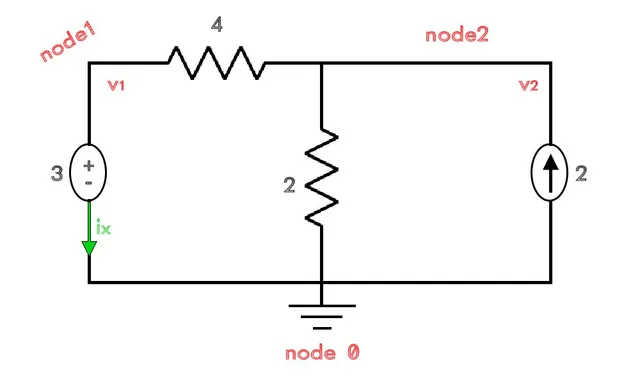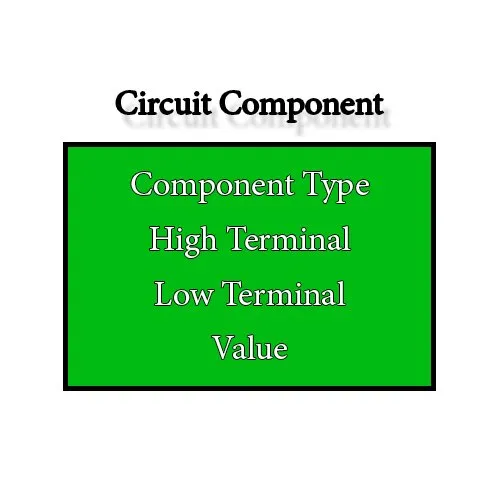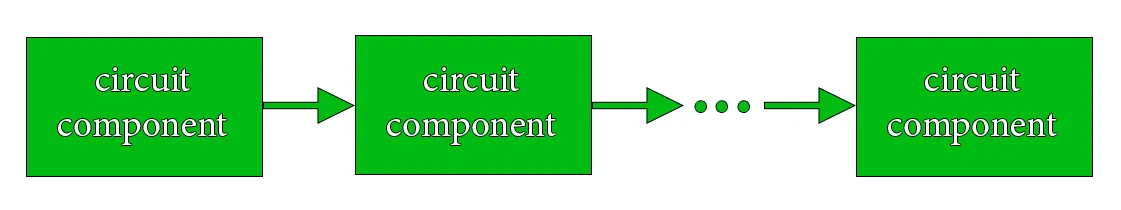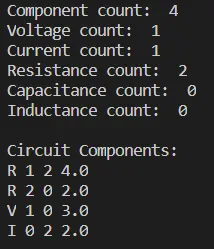# Electronic Circuit Simulation - Static Analysis Implementation (part 1) [Python][Custom Thumbnail]

All the Code of the series can be found at the Github repository:
https://github.com/drifter1/circuitsim

# Introduction

Hello it's a me again @drifter1! Today we continue with the Electronic Circuit Simulation series, a tutorial series where we will be implementing a full-on electronic circuit simulator (like SPICE) studying the whole concept and mainly physics behind it! In this article we start with the Implementation of a complete simulator for Static Analysis. The first step is processing the information of a Netlist and finding an efficient way of storing it to access the circuit information (components) later...

## Requirements:

• Physics and more specifically Electromagnetism Knowledge
• Knowing how to solve Linear Systems using Linear Algebra
• Some understanding of the Programming Language Python

## Difficulty:

Talking about the series in general this series can be rated:
Today's topic(s) can be rated:
• Intermediate

# Actual Tutorial Content

## Spice Netlist Format

A Spice Netlist is basically a text representation of an electronic circuit. It's an easy way of describing any electronic circuit using a specific and commonly known circuit description language or syntax. Such a file is organized into different sections:
• Model Description - electrical properties of particular devices
• Netlist Description - information about the various components
• Simulation Commands - simulation time, initialisation etc.

A Netlist is written in lines, where each line represents a model, component or command, except the first line which is the title of the simulation and most commonly being ignored. Of course any line that is left blank is also being ignored. We can also write comments by putting a '*' (asterisk) in the beginning of a line. To distinguish models, components and commands, the following conventions are used:
• Netlist descriptions start with letters that represent the various components and are followed by a number to distinguish the specific component (more into that later on)

In other words we can summarize everything as:
``````Simulation Title
*** Model Description ***
.model ...
*** Netlist Description ***
X1 ...
Y1 ...
*** Simulation Commands ***
.op
.end``````

Worth mentioning are also the following characteristics about Netlists:
• They are non-case-sensitive
• All tabs and spaces are dealt with like a single space
• The nodes are strings and '0' is always the ground node
• The component values are floating point numbers

### Simplification

In Static Analysis we only need to talk about 5 components and there also is no need to define specific electrical properties (models) about them. Let's also not forget that we don't need simulation commands in Static Analysis, as nothing really changes over time. We can't change a simulation parameter to calculate different currents or voltages. The value of those quantities clearly depends on the constant values of the various resistors and voltage/current sources. Capacitors and Inductors for now, are also only included for later stuff, and will not have such a great impact to our simulator...

So, how will our simplified Netlist look like? Well, we will simply ignore any line that starts with '*' (comment) or '.' (model or command) and only take care of the Netlist part which has to do with the components of an electronic circuit. The components are described as:
``````V<name> <+> <-> <value> → independent voltage source
I<name> <+> <-> <value> → independent current source
R<name> <+> <-> <value> → resistor
C<name> <+> <-> <value> → capacitor
L<name> <+> <-> <value> → inductor``````
where:
• X<name> is how we define the component type and its name
• <+> and <-> are the positive and negative terminals/nodes that the component is connected with. Those are strings
• <value> are the numerical values of the components in their corresponding units. Those are floating point numbers.

### Example Netlist

Let's consider the first example circuit from the previous article:In the Python part I wrote the circuit as:
``````+ ┌─R1 ┬────┐
V1   R2   I1 ↑
- └────┼────┘
⏚``````
which more clearly shows the names of the various components...

By using the names: '0', '1' and '2' for the nodes/terminals, we can write the circuit in Spice Netlist Format as:
``````R1 1 2 4
R2 2 0 2
V1 1 0 3
I1 0 2 2``````
Of course the Resistor terminals can be written in any order...as it doesn't change how they behave. But, we have to be careful in voltage and current sources.

## Reading and Storing the Components

Knowing the format of a Netlist we can easily read it, but where do we store this information? We of course have to define some structure that will store the information of a circuit component. More specifically, a circuit component can be represented by the following:### Component List

The information of each component is only needed once, as the "impact" to the MNA System can be done directly, but we don't know the total number of components of each group or the number of nodes that the circuit has from the beginning. So, we will have to store all the components in some structure. The easiest way to do this is using a list structure:In Python we will only have to define the Component structure/class, as the list is already one of the predefined aspects of that language. In other words, we only have to write:
``````class Component:  # circuit component structure
def __init__(self, comp_type, high_node, low_node, value):
self.comp_type = comp_type
self.high_node = high_node
self.low_node = low_node
self.value = value
def __repr__(self):
return str(self.comp_type) + " " + str(self.high_node) + " "
+ str(self.low_node) + " " + str(self.value)``````
To have an easy way to print a component we also redefine the representation function "__repr__()" to print out each entry separated with a single space character...

### Parsing Netlist

To parse the netlist we open up the file and loop through each line. Each of these lines will be split into 4 parts: component type, positive node, negative node, value. That can be done very easily using "split()". For the first entry we only need the first character. To make sure that this character is always upper-case we will use the string function "upper()". Similarly, we will use the same function to make sure that the string-nodes are also upper-case. Last but not least, the last entry will be converted into a floating point number using the function "float()". All these entries can then be appended to a components list...

So, in the end we have:
``````def parseFile(fileName):
file = open(fileName, "r")
# component list
components = []
for line in file:
# split line
parts = line.split()
components.append(
Component(parts.upper(), parts.upper(),
parts.upper(), float(parts)))
# return components
return components``````

### Counting Components

Something quite useful for the matrix creation will be the count of the components, as it will help us calculate the number of g2-components in the circuit, and so define the dimensions of the matrices. Therefore, we will define 5 variables, one for each component type that we have now, and will simply add an if-statement to the "parseFile()" function that updates those variables. To make sure that we don't create new local variables inside of the function we will also add a "global" scope management line to the function for those 5 variables. In the end we end up with the following updated version of "parseFile()":
``````def parseFile(fileName):
file = open(fileName, "r")
# use global scope variables for component counts
global voltageCount, currentCount, resistorCount, capacitorCount, inductorCount
# component list
components = []
for line in file:
# split line
parts = line.split()
components.append(
Component(parts.upper(), parts.upper(),
parts.upper(), float(parts)))
# update component counts
if parts == 'V':
voltageCount = voltageCount + 1
elif parts == 'I':
currentCount = currentCount + 1
elif parts == 'R':
resistorCount = resistorCount + 1
elif parts == 'C':
capacitorCount = capacitorCount + 1
elif parts == 'L':
inductorCount = inductorCount + 1
# return components
return components``````

### Main Function

To initialize the 5 count variables, call this simple parser and print out the information that we get, we have to define some very basic main function like this one:
``````# Initialize component counters
voltageCount = 0
currentCount = 0
resistorCount = 0
capacitorCount = 0
inductorCount = 0
# Parse File
fileName = "example.spice"
components = parseFile(fileName)
# Print Information
print("Component count: ", len(components))
print("Voltage count: ", voltageCount)
print("Current count: ", currentCount)
print("Resistance count: ", resistorCount)
print("Capacitance count: ", capacitorCount)
print("Inductance count: ", inductorCount)
print("\nCircuit Components:")
for i in range(0, len(components)):
print(components[i])``````

Using the Netlist file from the example, the output of the simulator so far is:Nothing special yet, but still parsing the information of a Netlist file is quite important, as this information will be used in the next steps of the simulator!

## RESOURCES

### References:

1. http://eee.guc.edu.eg/Courses/Electronics/ELCT503%20Semiconductors/Lab/spicehowto.pdf

Mathematical Equations were made using quicklatex

## Final words | Next up on the project

And this is actually it for today's post and I hope that you enjoyed it!

Next time we will continue with the Implementation of the Electronic Circuit Simulator for Static Analysis, showing how we map the nodes (low and high strings) into corresponding indexes of the MNA matrices...

So, see ya next time!

## GitHub Account:

https://github.com/drifter1Keep on drifting! ;)

H2
H3
H4
3 columns
2 columns
1 column